## Analyzing Control Systems with Delays

This example shows how to use Control System Toolbox™ to analyze and design control systems with delays.

### Control of Processes with Delays

Many processes involve dead times, also referred to as transport delays or time lags. Controlling such processes is challenging because delays cause linear phase shifts that limit the control bandwidth and affect closed-loop stability.

Using the state-space representation, you can create accurate open- or closed-loop models of control systems with delays and analyze their stability and performance without approximation. The state-space (SS) object automatically keeps track of "internal" delays when combining models, see the "Specifying Time Delays" tutorial for more details.

### Example: PI Control Loop with Dead Time

Consider the standard setpoint tracking loop: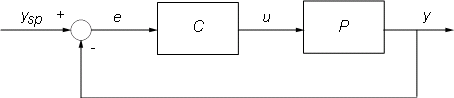where the process model `P` has a 2.6 second dead time and the compensator `C` is a PI controller:

``

You can specify these two transfer functions as

```s = tf('s'); P = exp(-2.6*s)*(s+3)/(s^2+0.3*s+1); C = 0.06 * (1 + 1/s); ```

To analyze the closed-loop response, construct a model `T` of the closed-loop transfer from `ysp` to `y`. Because there is a delay in this feedback loop, you must convert `P` and `C` to state space and use the state-space representation for analysis:

```T = feedback(P*C,1) ```
```T = A = x1 x2 x3 x1 -0.36 -1.24 -0.18 x2 1 0 0 x3 0 1 0 B = u1 x1 0.5 x2 0 x3 0 C = x1 x2 x3 y1 0.12 0.48 0.36 D = u1 y1 0 (values computed with all internal delays set to zero) Internal delays (seconds): 2.6 Continuous-time state-space model. ```

The result is a third-order model with an internal delay of 2.6 seconds. Internally, the state-space object `T` tracks how the delay is coupled with the remaining dynamics. This structural information is not visible to users, and the display above only gives the A,B,C,D values when the delay is set to zero.

Use the `STEP` command to plot the closed-loop step response from `ysp` to `y`:

```step(T) ```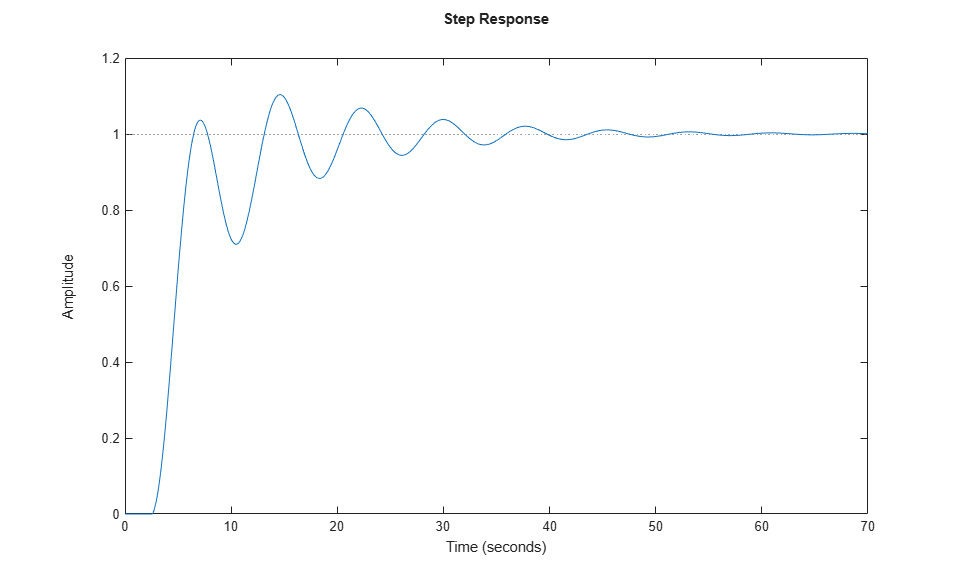The closed-loop oscillations are due to a weak gain margin as seen from the open-loop response `P*C`:

```margin(P*C) ```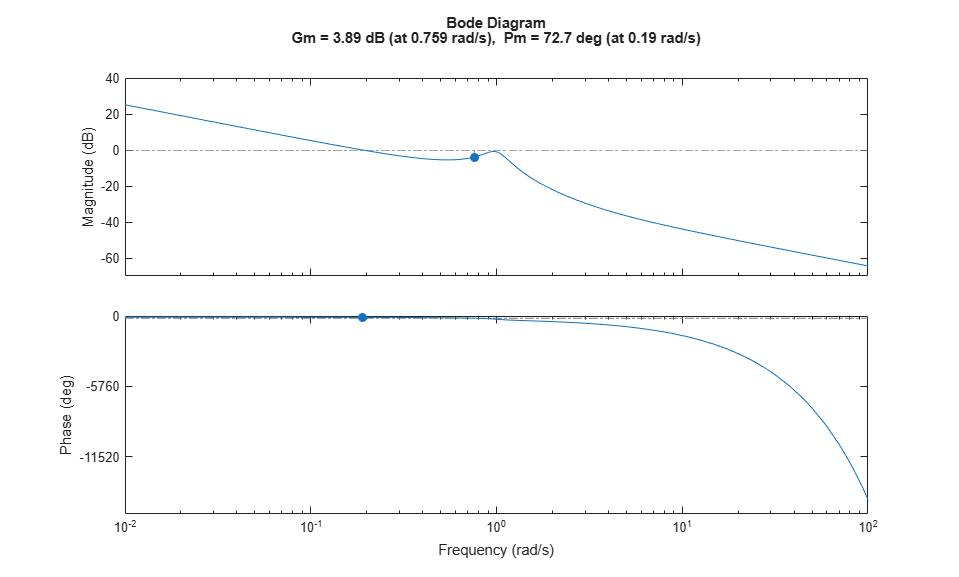There is also a resonance in the closed-loop frequency response:

```bode(T) grid, title('Closed-loop frequency response') ```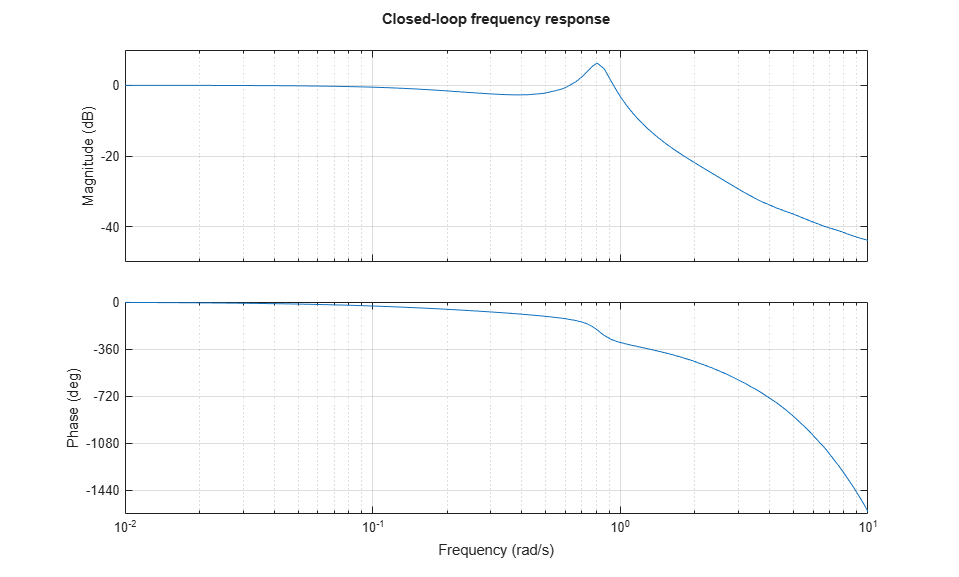To improve the design, you can try to notch out the resonance near 1 rad/s:

```notch = tf([1 0.2 1],[1 .8 1]); C = 0.05 * (1 + 1/s); Tnotch = feedback(P*C*notch,1); step(Tnotch), grid ```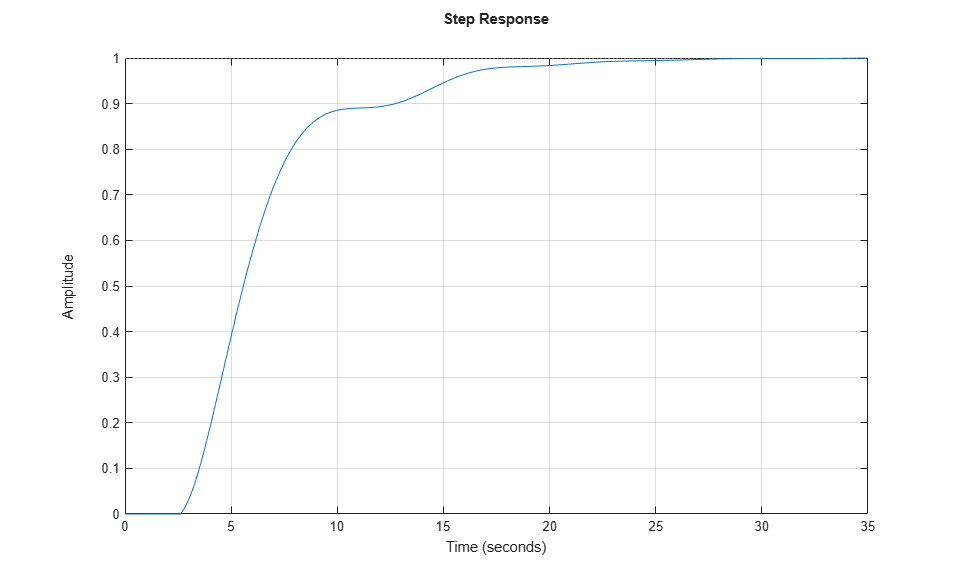### Pade Approximation of Time Delays

Many control design algorithms cannot handle time delays directly. A common workaround consists of replacing delays by their Pade approximations (all-pass filters). Because this approximation is only valid at low frequencies, it is important to compare the true and approximate responses to choose the right approximation order and check the approximation validity.

Use the `PADE` command to compute Pade approximations of LTI models with delays. For the PI control example above, you can compare the exact closed-loop response `T` with the response obtained for a first-order Pade approximation of the delay:

```T1 = pade(T,1); step(T,'b',T1,'r',100) grid, legend('Exact','First-Order Pade') ```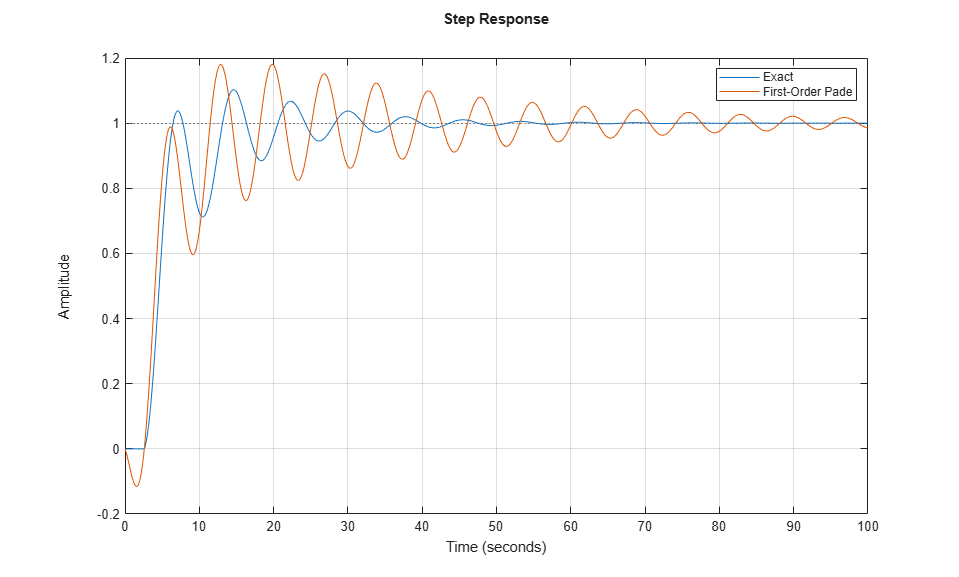The approximation error is fairly large. To get a better approximation, try a second-order Pade approximation of the delay:

```T2 = pade(T,2); step(T,'b',T2,'r',100) grid, legend('Exact','Second-Order Pade') ```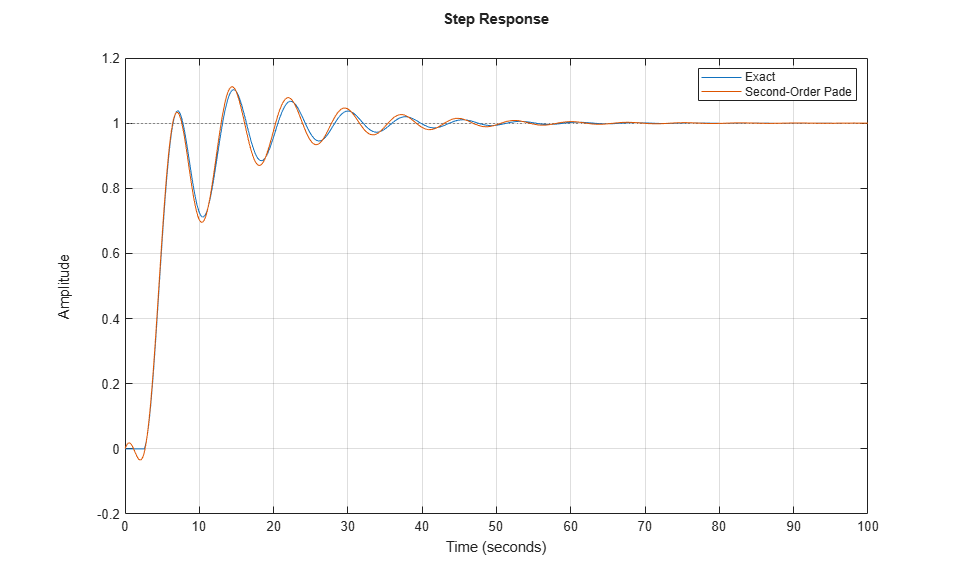The responses now match closely except for the non-minimum phase artifact introduced by the Pade approximation.

### Sensitivity Analysis

Delays are rarely known accurately, so it is often important to understand how sensitive a control system is to the delay value. Such sensitivity analysis is easily performed using LTI arrays and the InternalDelay property.

For example, to analyze the sensitivity of the notched PI control above, create 5 models with delay values ranging from 2.0 to 3.0:

```tau = linspace(2,3,5); % 5 delay values Tsens = repsys(Tnotch,[1 1 5]); % 5 copies of Tnotch for j=1:5 Tsens(:,:,j).InternalDelay = tau(j); % jth delay value -> jth model end ```

Then use `STEP` to create an envelope plot:

```step(Tsens) grid, title('Closed-loop response for 5 delay values between 2.0 and 3.0') ```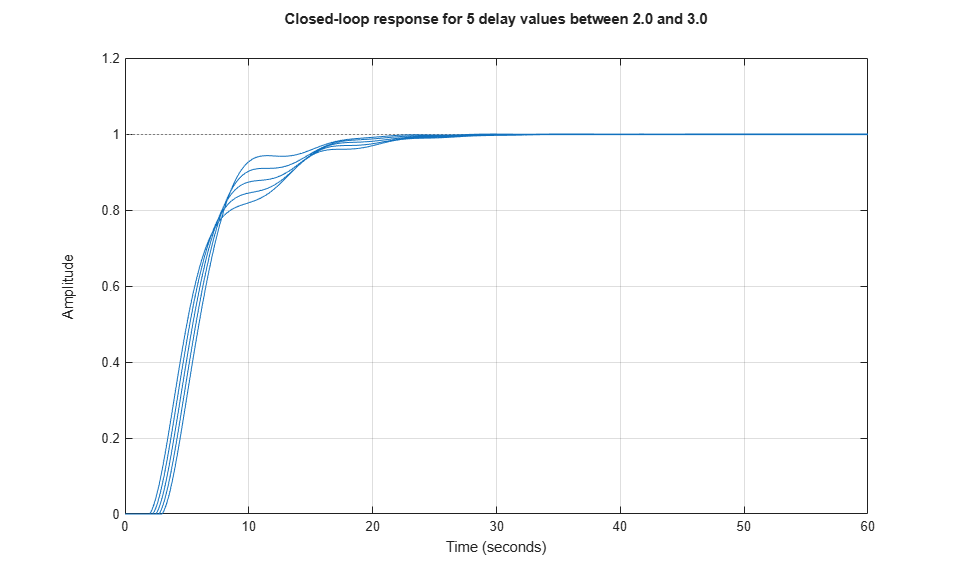This plot shows that uncertainty on the delay value has little effect on closed-loop characteristics. Note that while you can change the values of internal delays, you cannot change how many there are because this is part of the model structure. To eliminate some internal delays, set their value to zero or use `PADE` with order zero:

```Tnotch0 = Tnotch; Tnotch0.InternalDelay = 0; bode(Tnotch,'b',Tnotch0,'r',{1e-2,3}) grid, legend('Delay = 2.6','No delay','Location','SouthWest') ```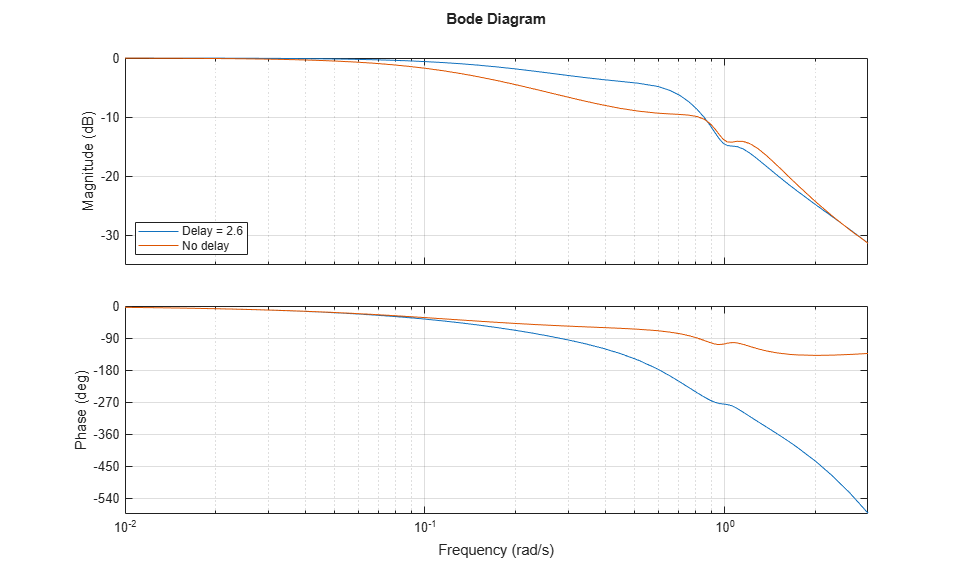### Discretization

You can use `C2D` to discretize continuous-time delay systems. Available methods include zero-order hold (ZOH), first-order hold (FOH), and Tustin. For models with internal delays, the ZOH discretization is not always "exact," i.e., the continuous and discretized step responses may not match:

```Td = c2d(T,1); step(T,'b',Td,'r') grid, legend('Continuous','ZOH Discretization') ```
```Warning: Discretization is only approximate due to internal delays. Use faster sampling rate if discretization error is large. ```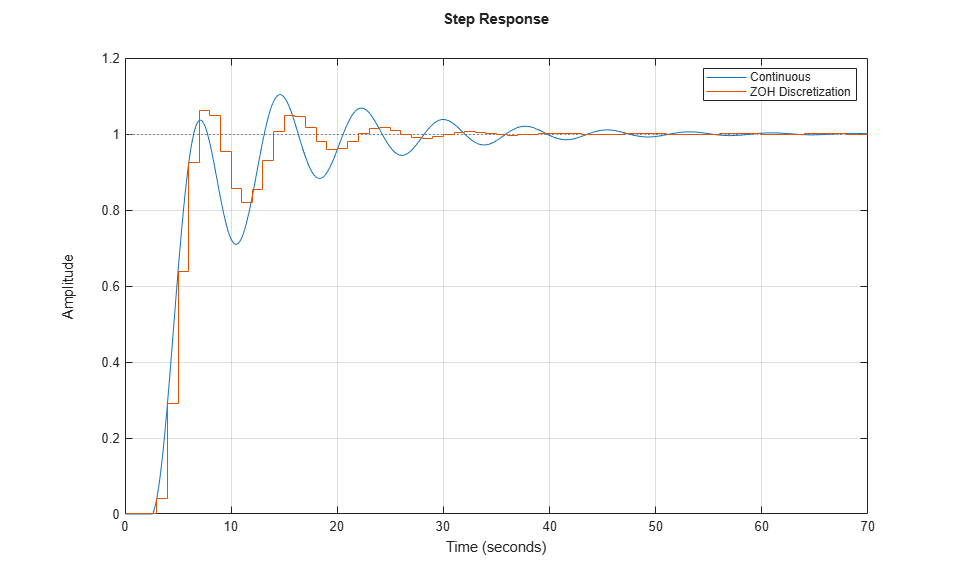To correct such discretization gaps, reduce the sampling period until the continuous and discrete responses match closely:

```Td = c2d(T,0.05); step(T,'b',Td,'r') grid, legend('Continuous','ZOH Discretization') ```
```Warning: Discretization is only approximate due to internal delays. Use faster sampling rate if discretization error is large. ```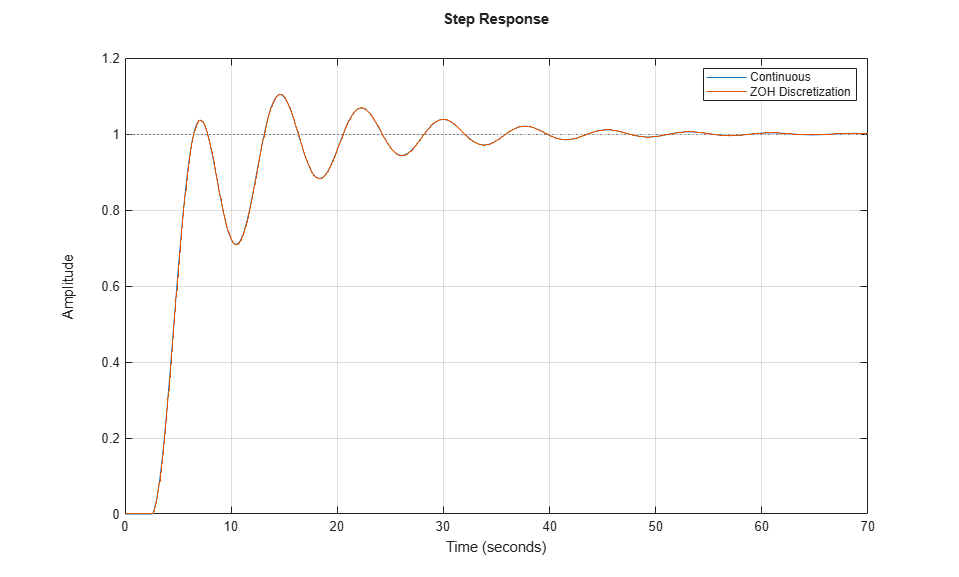Note that internal delays remain internal in the discretized model and do not inflate the model order:

```order(Td) Td.InternalDelay ```
```ans = 3 ans = 52 ```

### Some Unique Features of Delay Systems

The time and frequency responses of delay systems can look bizarre and suspicious to those only familiar with delay-free LTI analysis. Time responses can behave chaotically, Bode plots can exhibit gain oscillations, etc. These are not software quirks but real features of such systems. Below are a few illustrations of these phenomena

Gain ripples:

```G = exp(-5*s)/(s+1); T = feedback(G,.5); bodemag(T) ```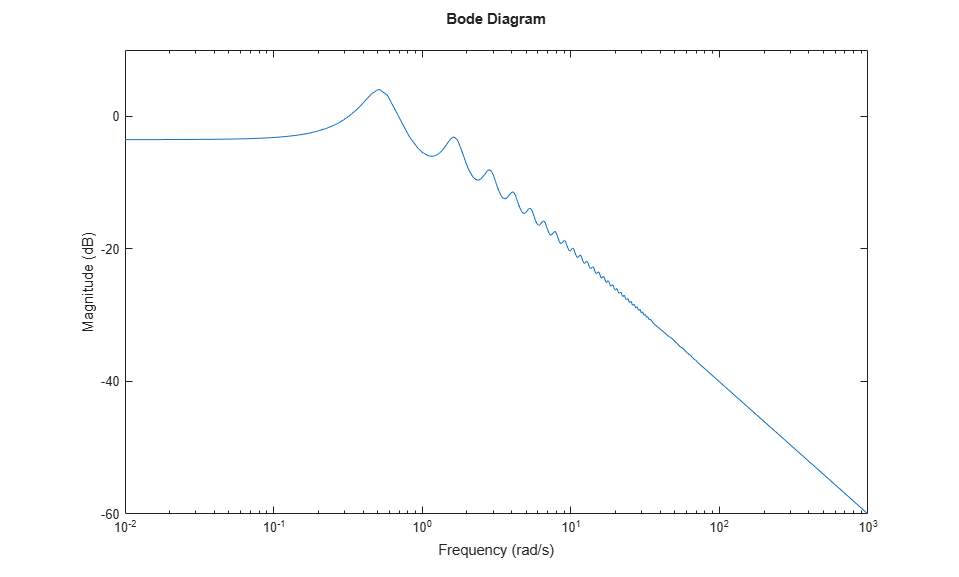Gain oscillations:

```G = 1 + 0.5 * exp(-3*s); bodemag(G) ```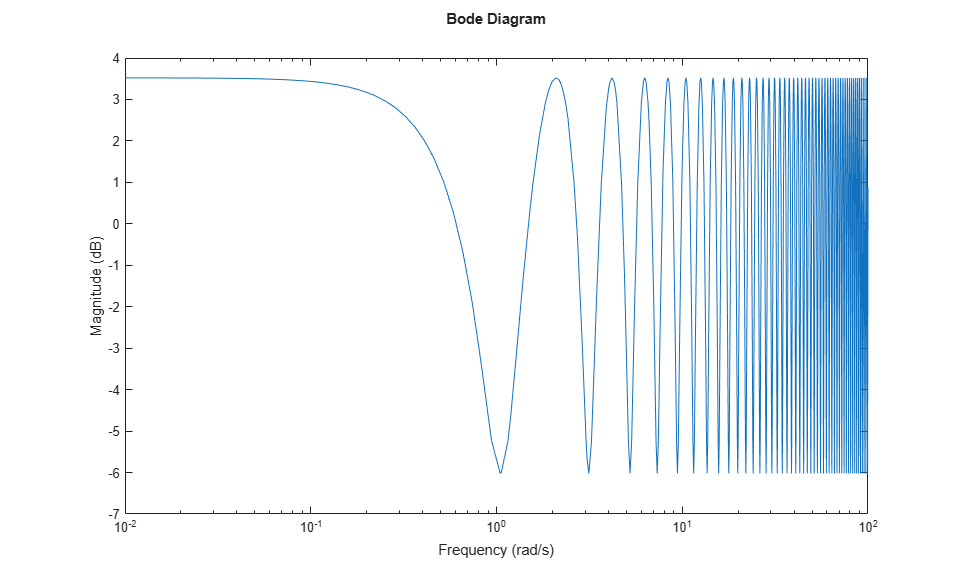Jagged step response (note the "echoes" of the initial step):

```G = exp(-s) * (0.8*s^2+s+2)/(s^2+s); T = feedback(G,1); step(T) ```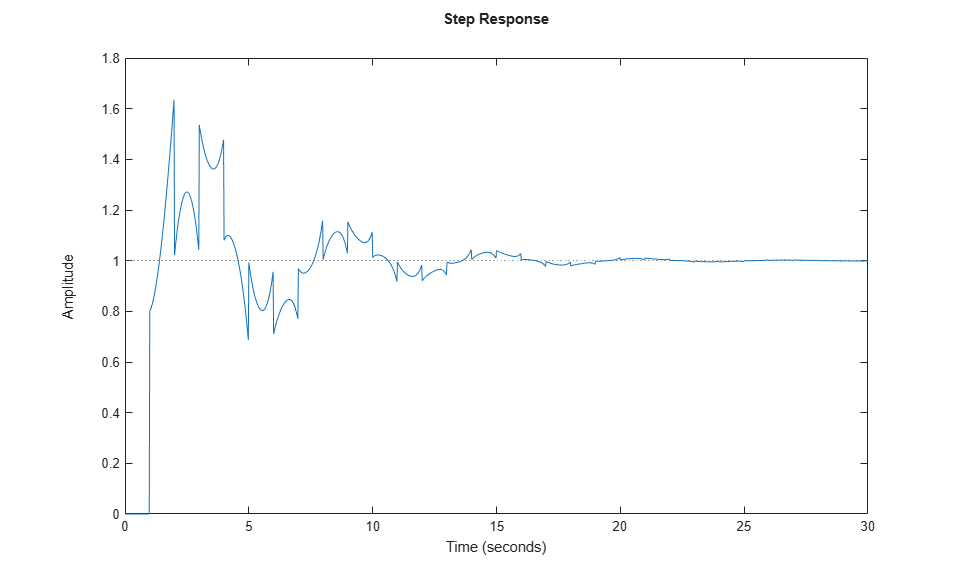Chaotic response:

```G = 1/(s+1) + exp(-4*s); T = feedback(1,G); step(T) ```